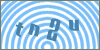Products
ServicesScripts: Javascript :: Html5 :: Library Article #32Developer's SectionCreating a 3D World Using the Canvas Element and the CanvasX or Three.js Library
By: Erobo Software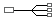Hire a Developer for Related Work / Installation | \$55 hr
 Rating: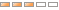| Rate It: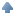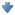Average Votes: (3314) Favorites:This article describes a technique to create a virtual world in 3D using the new HTML5 Canvas Element and the CanvasX / Three.js library. The article discusses basic terrain layout and object positioning.

It's very easy to create a virtual world using the HTML5 Canvas element along with the CanvasX or three.js library. These libraries come bundled with a renderer that can either output the virtual world using WEBGL (Which sometimes requires plug-in activation) or the Canvas element which is generally enabled by all modern web browsers. In the following demo we will try to recreate the main elements of a virtual world such as the one on (http://www.virtual3ds.com/erobo_software) which consist of the sky, terrain, and mountains:

Now, let's take a look at the code: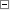Code Snippet 1<!-- Declare the container where we stick the canvas-->
<center>
<div id="mycontainer"></div>
</center>
<!-- Include Three.js Library-->
<script language="javascript" type="text/javascript"
src="https://www.erobo.net/scripts/javascript/32/scripts/CanvasX.min.js"></script>

<script language="javascript">
//get reference to the container
var container = document.getElementById('mycontainer');
//declare one texture
var texture = new THREE.Texture( container );
var material_1 = new THREE.MeshBasicMaterial( { map: texture, overdraw: true } );
var material_2 = new THREE.MeshPhongMaterial( { color:0x90EE90, overdraw: true } );

var textureImgSky = new Image();
texture.needsUpdate = true;
material_1.map.image = this;
start3dWorld();
}
};

textureImgSky.src = '32/scripts/virtual_world_sky.gif';

// create the sun's material
var sphereMaterial = new THREE.MeshBasicMaterial(
{
color: 0xF7E039,
shininess: 0.8
});

function start3dWorld(){

// set the scene size
var WIDTH = 464,
HEIGHT = 380;

// set some camera attributes
var VIEW_ANGLE = 45,
ASPECT = WIDTH / HEIGHT,
NEAR = 0.1,
FAR = 7000;

// create a canvas renderer, camera
// and a scene
var camera = new THREE.PerspectiveCamera(VIEW_ANGLE,
ASPECT,
NEAR,
FAR);
var scene = new THREE.Scene();

// the camera starts at 0,0,0 so pull it back
camera.position.z = 300;
camera.position.y = 0;
camera.position.x = 0;

var vw_matrix = new THREE.Matrix4();
//create sky geometry
var geometry_sky = new THREE.PlaneGeometry(1900, 1900);

geometry_sky.applyMatrix(vw_matrix);
geometry_sky.computeFaceNormals();
geometry_sky.computeVertexNormals();
geometry_sky.computeTangents();

//create sky plane set behind the objects
var plane = new THREE.Mesh(geometry_sky,
material_1);
plane.overdraw = true;
plane.position.set(0, 0, -1500);

//create the terrain
var geometry_terrain = new THREE.PlaneGeometry(1500, 1500, 30, 30);

geometry_terrain.applyMatrix(vw_matrix);

//indicate this terrain is subject to change
geometry_terrain.dynamic = true;
geometry_terrain.computeFaceNormals();
geometry_terrain.computeVertexNormals();
geometry_terrain.computeTangents();

geometry_terrain.vertices.z = 35;
geometry_terrain.vertices.z = 100;
geometry_terrain.vertices.z = 35;
geometry_terrain.vertices.z = 35;
geometry_terrain.vertices.z = 100;
geometry_terrain.vertices.z = 35;
geometry_terrain.vertices.z = 35;
geometry_terrain.vertices.z = 60;
geometry_terrain.vertices.z = 90;
geometry_terrain.vertices.z = 120;
geometry_terrain.vertices.z = 150;
geometry_terrain.vertices.z = 120;
geometry_terrain.vertices.z = 90;
geometry_terrain.vertices.z = 60;
geometry_terrain.vertices.z = 35;

//vertices are dirty so indicate
geometry_terrain.__dirtyVertices = true;
geometry_terrain.computeVertexNormals();

//create terrain plain
var plane2 = new THREE.Mesh(geometry_terrain,
material_2);
plane2.overdraw = true;
plane2.position.set(-3, -200, -750);
//bring the plain down into a horizontal position
plane2.rotation.x = - (Math.PI / 2);

// set up the sun vars
var radius = 50, segments = 10, rings = 10;

//create inner hot core
var sphere2 = new THREE.Mesh(
sphereMaterial);
sphere2.position.set(-250, 220, -600);

var point_light = new THREE.PointLight(0xFFFFFF);
point_light.position.x = -50;
point_light.position.y = 500;
point_light.position.z = -850;
point_light.intensity = 1.0;

//create renderer
var renderer = new THREE.CanvasRenderer();

// start the renderer
renderer.setSize(WIDTH, HEIGHT);

// attach the render-supplied DOM element
container.appendChild(renderer.domElement);

renderer.render(scene, camera);
}

</script>

Additionally, when you want to add more complex objects with images to your 3D world, you can use a 3D vector drawing application that can assist you with plotting your 3D points (x,y,z). These type of apps can therefore save you tons of work by automatically creating the geometries, faces, and uvs and do the export to the web and/or CanvasX.js / Three.js. You can download 3D Vector Drawer developed by Erobo to help you create your own virtual world in a convenient way.

And that's the end, enjoy.See other Scripts in Html5Submit Your Scripts:If you would like to have your Javascripts published in this section please fill outthe form below:
 [ Refresh Image ]